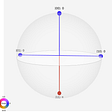# Why all of the fuzziness?

1. Arcane conceptual basis of quantum mechanics itself.
2. Much material was sourced from or for physicists and mathematicians, not classical computer scientists.
3. The difficulty of linear algebra, especially how it meshes with real physical systems.
4. Terminology that is needlessly inconsistent with classical computing. Operations or instructions on a quantum computer are called “gates”, which is classically a hardware term. A quantum program is referred to as a “circuit”, which is classically a physically-connected collection of hardware components rather than the sequence of operations which comprise a classical program. A qubit refers to physical hardware, comparable to a flip flop, in contrast to a classical bit which is information rather than a hardware device — which holds a bit.
5. Quantum computing has not yet developed its own level of abstraction to remove it from the realm of physics, arcane mathematics, and discrete electronic devices (e.g., transistors, digital logic gates, and flip flops), comparable to how classical computing has developed with Boolean logic, Turing machines, and computer science in general. Need for development of formalisms for a quantum computer science.
6. The probabilistic nature of quantum computing requires a different mindset than the hard and reliable determinism of classical computing.
7. Lack of clarity, specificity, and detail of terminology of quantum computing, beyond the difficulties with quantum mechanics and linear algebra.
8. Vagueness in specification of operations (gates) in quantum programs.
9. Lack of detail for specification for edge cases for quantum operations.
10. Lack of detail for parameters of quantum operations which are continuous rather than discrete 0 or 1.
11. A little too much hand-waving. Okay, way too much hand-waving.
12. More than a little too much hype. Too much marketing when more basic science is needed.
13. Significant intuition is needed, but the intuition is distinctly unintuitive. Much better guidance is needed for developing the requisite intuition.
14. Insufficient guidance for design of algorithms.
15. Insufficient guidance and detail for basic building blocks for algorithms.
16. Lack of consistency between the structure of documentation for quantum computers from different vendors.
17. Severe hardware limitations of existing and near-term quantum computers make it very difficult to conceptualize practical solutions to practical problems that have a chance of running on current and near-term real quantum computers.

# The more general obstacles

1. Bloch sphere is too simplistic and incomplete an account of how qubits really function. Amplitude is not represented. Orthonormal nature of basis vectors is not represented. Joint state is not represented, such as a register of qubits which are each superimposed using a Hadamard gate but not entangled.
2. Programming a quantum computer is comparable to machine or assembly language on a classical computer, but even more primitive, with no notion of even basic arithmetic or the features of a Turing machine, and lacking higher-level language abstractions such as functions with parameters, conditional branching, looping, and rich data types (including text, images, and media data.) Developing software or designing algorithms for a quantum computer is like working with raw transistors, simple digital logic gates, and bare flip flops.
3. How does entanglement factor into design of algorithms? The question is not how to entangle two qubits, but how generally to exploit entanglement in algorithms. To put it more plainly, what exactly is entanglement good for, and how should it be used? How does entanglement work in a typical algorithm? Need for explicit design principles and design patterns.
4. How does interference factor into design of algorithms? Similar issues as with entanglement. How does interference work in a typical algorithm? Need for explicit design principles and design patterns.
5. How does quantum parallelism factor into design of algorithms? Similar issues as with entanglement and interference. How does quantum parallelism work in a typical algorithm? Need for explicit design principles and design patterns. A lot of the steps are fairly straightforward, but there are some unintuitive intuitive leaps as well. The unintuitive intuition needs more detailed elaboration with less hand waving. A lot of the treatments explicitly admit that you have to be quite clever at extracting usable (classical) data from the results of a quantum parallel computation, but offer little explanation of how to arrive at that cleverness.
6. How does phase estimation factor into design of algorithms? Need for explicit design principles and design patterns.
7. How does phase in general factor into design of algorithms? Need for explicit design principles and design patterns.
8. How exactly does amplitude amplification work, and how is it factored into the design of algorithms? Referenced a few places, but sketchy on details, let alone more general guidance with principles and design patterns.
9. Are rotations about the three axes inherently analog and continuous rather than digital or quantized? In contrast to the binary nature of the two basis states of |0> and |1>.
10. Why must all quantum computation necessarily be reversible? Okay, quantum mechanics says so, but… why? What is the actual phenomenon at work here?
11. Why does measurement necessarily result in collapse of the wave function? Okay, quantum mechanics says so, but… why? What is the actual phenomenon at work here?
12. Why is the no-cloning theorem necessarily true? Again, okay, quantum mechanics says so, but… why? What is the actual phenomenon at work here? And how can the SWAP gate work at all if the no-cloning theorem is true?
13. It is difficult to mentally conceptualize how to factor quantum decoherence into the design of quantum algorithms beyond toy-like size. Such as with Shor’s algorithm where millions of gates will have to be executed for factoring 2048 and 4096-bit integers.
14. It is difficult to mentally conceptualize the probabilistic nature of quantum parallelism into the design of quantum algorithms beyond toy-like size. Such as with Shor’s algorithm where modular exponentiation will be performed on 2048 and 4096-bit integers — and deterministic results are needed.
15. Relevance of number theory to quantum computation, such as order-finding and Shor’s algorithm, and phase estimation. It’s too much to expect algorithm designers to be expert in the arcane mathematics of number theory, so a customized, sanitized subset as well as principles and design patterns focused on quantum algorithms are needed. Not just the magic formulas to be used, but guidance for developing an intuition for how to integrate number theory and quantum computation.
16. Need for a lot more examples of quantum computing concepts being applied to real-world problems, with detailed plain English narrative about how the quantum computing concepts are actually being used and how they actually work.
17. Why exactly does a Clifford gate set plus CNOT constitutes a universal gate set? Universal with respect to what? Plain language, please.
18. What computational tasks are possible using quantum computing? Current descriptions are rather minimal at best.
19. Need for a richer set of quantum algorithmic building blocks, rather than simply raw gates and a few larger algorithms such as QFT and phase estimation. With both their external function and their internal operation explained fully in detail in plain language.
20. Need for a plain language narrative design guide for how to convert plain language real-world problems into quantum solutions using quantum algorithmic building blocks.
21. Quantum computing is infected with too many Greek symbols and odd notations inherited from quantum mechanics and mathematics which make it much more difficult to read and certainly more difficult to write. Simple symbolic names are needed for most constructs.
22. More online interactive quantum simulators are needed, both for real machines and for general, abstract, theoretical quantum computers. They should display all amplitude transitions, even though amplitudes are not observable in real quantum computers.
23. More online interactive quantum code repositories (GitHub) are needed for common gate sequences. And easy to access them within online interactive simulators.
24. Detailed comments are needed for all quantum code. Detail why the code is doing what it is doing, especially any interesting or unexpected consequences or side effects. Include links to any online academic papers from which the gate sequences were derived.
25. Need for more formalized specification and documentation for the programming model for quantum computers, both in general and for each specific model of machine. Focusing on the instruction set, gates, or operations. Everything which a quantum programmer needs to know to successfully develop a quantum program, without the need for experimental trial and error just to figure out what operations (gates) actually do. See my Framework for Principles of Operation for a Quantum Computer paper for a proposal for the content of such a document. Current documentation is vague, imprecise, and incomplete.
26. Even with relatively complete documentation of the programming model, it may still be necessary to have detailed documentation of the implementation of the programming model to gain enough insight to develop a true intuition for programming a particular quantum computer or even quantum programming in general. See the Implementation Specification section of my Framework for Principles of Operation for a Quantum Computer paper for a proposal for the content of such a document.
27. Even with relatively complete documentation of the programming model and its implementation, it may still be necessary to have access to the source code for the firmware and possibly even the schematics for the electronics of the quantum computer, including any configuration parameter settings, to gain enough insight to develop a true intuition for programming a particular quantum computer or even quantum programming in general. But, the theory is that the documentation — principles of operation and implementation — should be sufficient and have all the necessary hints to develop the necessary intuition. Still, a fully open source quantum computer would be a very good thing.
28. Being probabilistic, how close to deterministic solutions can we expect from quantum computing? How does this constrain or limit what problems or algorithms are appropriate for quantum computing? Alternatively, to what extent have we been fooling ourselves all along when accepting strictly deterministic solutions from classical computing for real-world problems which are inherently probabilistic rather than strictly deterministic?
29. How much depth of knowledge is needed about quantum information theory?
30. We need to devise a new set of terminology and language constructs for computer science that facilitate discussion of quantum computing in the context of a hybrid of classical and quantum computing. We need semantics for one world of computing, not two independent worlds. We need both highly technical terms and plain, natural language constructs as well. For example, “quantum parallelism” is fine as far as it goes as a simple, generic, abstract, umbrella term, which dead ends at a semantic cliff with no further plain language, non-jargon support.

# The more specific obstacles

1. How can quantum parallelism work for an n-qubit “register” if those input qubits are not entangled in some way? The Hadamard gates on each qubit created a superposition within each qubit, but not entanglement between qubits.
2. What is the simplest (and useful) example of quantum parallelism? With a full and detailed plain language explanation of how entanglement, interference, and other quantum computing concepts are being used. Most treatments skip over too many essential details — leaving them as “an exercise for the reader.”
3. Can more than two qubits be entangled — on current machines? So-called multipartite entanglement.
4. Can the |GHZ> and |W> states be constructed — on current machines, using only pairwise, bipartite entanglement gates?
5. Can CNOT gates be daisy-chained after an H gate to entangle more than two qubits — on current machines?
6. Which unitary transform formulations necessarily result in entanglement? What is the rule or magic than causes entanglement in some cases but not others?
7. Which gates necessarily result in entanglement? Only CNOT, or others as well?
8. Can some gates conditionally result in entanglement? Why? What is the underlying phenomenological mechanism at work?
9. What is the number of binary bits or decimal digits of precision supported for entries of unitary matrices and gate parameters, such as rotation and phase? Single-precision floating point? Double? Quad precision? What does the software API support, what does the firmware support, what does the gate execution support, what do the lasers, microwaves or other qubit manipulation technology support, and what do the actual qubits support?
10. What is the finest resolution of rotation for rotation gates? What is the smallest possible rotation? What is the largest possible rotation? What is the smallest possible difference between two rotations which can have a discernible impact on the results? Are rotations inherently analog rather than digital — continuous with a large number of values vs. discrete with a relatively small number of values? Is there any reason to think of rotations as digital rather than analog — other than rotation angles of multiples of pi/2? How many unique angles can be represented for each of the three axes? How many unique pairs of angles can be represented by simultaneous rotations of two axes? How many distinct states could a qubit be in?
11. Is the precision supported in unitary matrices and gate parameters sufficient for reasonably large quantum Fourier transforms (QFT) and phase estimation, such as is needed for using Shor’s algorithm to factor large integers, such as for 1024, 2048, and 4096-bit public encryption keys?
12. How does the SWAP gate avoid the no-cloning theorem?
13. If the two qubits for a SWAP gate are entangled with two other qubits, what exactly happens with those other two qubits? Sequence: entangle 1 and 2, entangle 3 and 4, now try to swap 2 and 3.
14. If the two qubits for a SWAP gate were entangled with each other, what exactly happens? Does anything change at all? It should be possible to answer this question purely from reading the doc for the SWAP gate. Or from the doc for CNOT if SWAP simply expands to three consecutive CNOT gates.
15. What happens if the target qubit of a CNOT gate is in a superimposed state already — it’s not exactly 1.0|0> or 1.0|1>? What if the magnitude of the amplitudes of the two basis states of the target qubit are unequal (and non-zero), such as sqrt(0.75)|0> + sqrt(0.25)|1>?
16. What happens if CNOT is performed on two qubits which are already entangled with two other qubits? Are all four qubits then entangled? Do the other two qubits become unentangled? Sequence: entangle 1 and 2, entangle 3 and 4, now try to entangle 2 and 3. And what happens if this last step was replaced with trying to entangle 1 and 4? Again, this needs to be clear from the doc — either or both the CNOT and entanglement doc.
17. What is the physical realization of phase? How discrete/quantum is it, or is it more analog? What resolution of rotation can be realized at the hardware/physics level, as distinct from what the machine is designed to provide to the programmer?
18. Why are there no Bell states with complex amplitudes? Or at least no examples, that I have seen.
19. What are mixed and pure states? Are there more than one meaning for these terms? Are the terms reserved only for multi-qubit ensembles, or can they apply to the two basis states of a single qubit?
20. If a state of |0> or |1> has an amplitude of 1.0, is it considered a pure state, or is that a misuse of the term, reserving it for multi-qubit ensembles? If pure state is not the proper term, what is the proper and preferred term?
21. Are joint states real, or just an artificial mental device? Such as performing a Hadamard transform on n qubits.
22. What is the smallest possible amplitude? If I have 1024 qubits and perform a Hadamard transform on all of them is the amplitude of each of the 2¹⁰²⁴ computational basis states of the joint state really 1/(2¹⁰²⁴), which seems too impossibly small to be real?
23. What exactly does it mean to measure in other than the computational basis? When might this be useful? More plain language and more examples are needed.
24. How is amplitude of the basis vectors represented in the Bloch sphere?
25. What exactly does a CNOT gate do if amplitude of |1> in control or target qubits is not exactly 0.0, 1.0, or sqrt(2)? Most descriptions simply refer to “if the control qubit is |1>” without describing what happens when a superposition with amplitude other than 1.0 or 0.0 is used.
26. What is a density matrix or density operator and how is it used or required in quantum computation?
27. What is the significance of the trace of a unitary matrix? How should programmers be using the concept in algorithm design? It’s simply defined as the sum of the entries on the main diagonal of a square matrix, but phenomenologically, what is actually going on and why is it significant? It has something to do with a density matrix and pure vs. mixed states. A more clear definition of pure and mixed states might clarify the matter.
28. How does Shor’s algorithm really work, or how well does it work? I have more detailed questions in my Some Preliminary Questions About Shor’s Algorithm for Cracking Strong Encryption Using a Quantum Computer paper.
29. How does quantum parallelism actually work? The technical details, but at a computational level rather than (and in addition to the) raw physics and raw quantum mechanics.
30. How does interference actually work? The technical details, but at a computational level rather than just the raw physics and raw quantum mechanics.
31. How is it that amplitude cannot be directly measured, but phase can be estimated?
32. How does phase estimation really work? If you can design a clever algorithm to estimate phase, why not just encode that algorithm in hardware or firmware and offer it as a gate, possibly with some parameters, including how many bits of precision you want, and then say that this pseudo-gate can measure phase (otherwise part of amplitude, which cannot be directly measured)?
33. How does a Fourier transform (quantum or non-quantum) actually work? What are the exponentials actually doing — in plain language? What is the intuition needed to feel comfortable with quantum Fourier transforms?
34. How much precision is needed for a quantum Fourier transform to be useful? What considerations and factors are relevant to the algorithm designer?
35. How much resolution is needed for a typical QFT? What factors need to be considered by the algorithm designer?
36. How restrictive can banding or other forms of approximate Fourier transform be without resulting in such an extreme loss of precision so as to render them relatively useless for other than mere toy examples?
37. There is disagreement between various treatments as to whether the exponent for a QFT is positive or negative. And hence, whether an inverse QFT has a negative or positive exponent. A classical DFT has a negative exponent, and its inverse has a positive exponent, but it seems common for treatments to claim that a QFT has a positive exponent. Maybe they are actually using an inverse QFT but neglecting the adjective. It feels too loose and sloppy to me. I see this treatment in Shor’s paper.
38. Is the divisor in the exponent of a QFT the number of bits or the number of states superimposed in those bits — n vs. 2^n?
39. How much do we need to know about Clifford groups and Clifford gates to adequately exploit quantum computing? Again, ALL in plain language — the raw mathematics is completely unhelpful.
40. What exactly would a continued fraction expansion look like? I’ve seen a couple of references, particularly for Shor’s algorithm, but no actual code. Presumably, this would be classical code, but what exactly would the quantum input look like?
41. Specific meaning of the phrases “up to a factor”, “up to a global phase”, and “up to a global phase factor”. Again, in plain language.
42. Is there a connection between period-finding (or order-finding) and phase estimation? Phase estimation is thrown around a little too loosely to be very helpful.
43. It would be helpful to give an intuitive, plain language justification for the need for complex numbers for amplitudes. Just because quantum mechanics uses them is not a sufficient justification. Maybe physicists and mathematicians are comfortable with them, but that’s hardly a justification for their use in quantum computing. Again, plain language.
44. It would be helpful to give an intuitive, plain language justification for the need for phase. I suspect that phase is one of the features of quantum computing which has a lot of untapped potential, but it’s still a bit too vague to know for sure. It hasn’t been presented well. Again, plain language is needed.
45. What operations can be performed on entangled qubits without disrupting (collapsing) their entanglement? It would be helpful to have an intuitive, plain language enumeration and explanation of the utility of various ways of working with entangled qubits.
46. Is there an explicit way to unentangle two (or more) qubits? I’ve seen no mention of this anywhere. And what state does it leave each qubit in?
47. Need a clear definition, explanation, discussion, and examples for global property. Including how it relates to interference and quantum parallelism. Again, plain language.
48. The concept of uncomputation needs more thorough treatment. Related to the concept of all quantum computation needing to be reversible. Seems to be more narrowly related to the reuse of ancillary qubits. The claim is that without it, interference needed for quantum computation will be disrupted. But, it’s all a bit too vague. Maybe a more thorough treatment of interference will shed some light. In particular, it’s unclear why uncomputation won’t be just as disruptive to interference as raw reuse of ancillary qubits.
49. How much of a deep knowledge of linear algebra is needed to fully comprehend and fully exploit quantum computation?
50. How much of a deep knowledge of Hilbert spaces is essential to fully comprehend and fully exploit quantum computation? Or vector spaces in general?
51. How much of a deep knowledge of complex numbers is needed to fully comprehend and fully exploit quantum computation?
52. How much of a deep knowledge of adjoint operators is needed to fully comprehend and fully exploit quantum computation?
53. How much of a deep knowledge of unitary matrices and unitary operators is needed to fully comprehend and fully exploit quantum computation?
54. How much of a deep knowledge of Hermitian operators is needed to fully comprehend and fully exploit quantum computation?
55. Is there any Bloch sphere-like visual model for Bell states?
56. Are there Bell states for entanglement of more than two qubits? There are also the |GHZ> and |W> states — are they technically “Bell” states or not?
57. How much of a deep knowledge of Ising coupling is needed to fully comprehend and fully exploit quantum computation?
58. How much of a deep knowledge of spin is needed to fully comprehend and fully exploit quantum computation?
59. How much depth of knowledge is needed about Hamiltonians?
60. Is a deep understanding of the wave-particle duality of quantum mechanics necessary to fully comprehend and fully exploit quantum computation? When are wave aspects more helpful and when are particle aspects more helpful?
61. How much depth of knowledge of wave functions is needed to fully comprehend and fully exploit quantum computation?

# What’s next?

--

--

--

## More from Jack Krupansky

Freelance Consultant

Love podcasts or audiobooks? Learn on the go with our new app.

## Threat of Bioaccumulation: New Study Investigates Impact of Polycyclic Aromatic Hydrocarbons on Bay…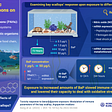## Spotting Cows from Space## Title: A brief history of time.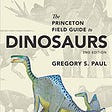## The Future of Search… and the Curious Machine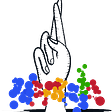## Why do electronic devices fail?## EBV-Positive B-Cell Lymphoma with Extensive Plasmacytic Differentiation & Increased CD30-Positive…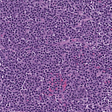## Jack Krupansky

Freelance Consultant

## An introduction to Quantum Computing